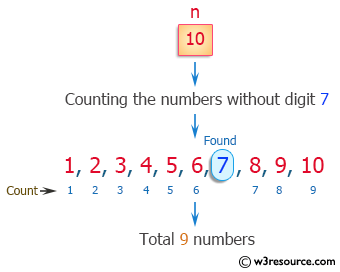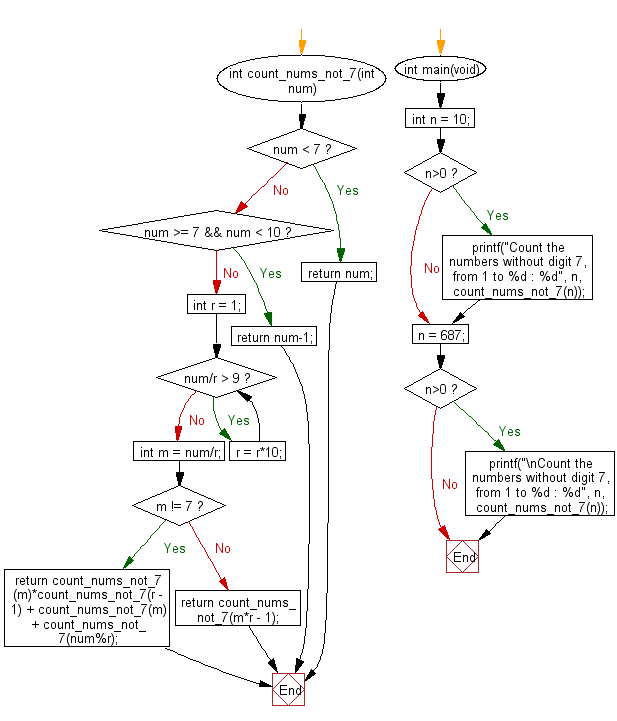﻿ C Program: Numbers without digit 7 from 1 to n

# C Exercises: Count the numbers without digit 7, from 1 to a given number

## C Programming Mathematics: Exercise-22 with Solution

Write a C program to count the numbers without the digit 7, from 1 to a given number.

Example 1:
Input: n = 10
Output: 9
Example 2:
Input: n = 687
Output: 555

Pictorial Presentation:Sample Solution:

C Code:

``````#include <stdio.h>
#include <stdlib.h>

int count_nums_not_7(int num)
{
if (num < 7)
return num;
if (num >= 7 && num < 10)
return num-1;

int r = 1;
while (num/r > 9)
r = r*10;

int m = num/r;

if (m != 7)
return count_nums_not_7(m)*count_nums_not_7(r - 1) + count_nums_not_7(m) + count_nums_not_7(num%r);
else
return count_nums_not_7(m*r - 1);
}
int main(void)
{
int n = 10;
if (n>0)
printf("Count the numbers without digit 7, from 1 to %d : %d", n, count_nums_not_7(n));
n = 687;
if (n>0)
printf("\nCount the numbers without digit 7, from 1 to %d : %d", n, count_nums_not_7(n));
}
```
```

Sample Output:

```Count the numbers without digit 7, from 1 to 10 : 9
Count the numbers without digit 7, from 1 to 687 : 555
```

Flowchart:C Programming Code Editor:

Improve this sample solution and post your code through Disqus.

What is the difficulty level of this exercise?

Test your Programming skills with w3resource's quiz.

﻿